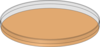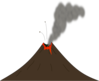Lower Elementary:
Question: Grace plants a pea for her science fair project and measures how much it grows each week. The first week, it grows 2 inches. After 2 weeks, it is 5 inches tall. After 3 weeks, it is 9 inches tall. After 4 weeks, it is 14 inches tall. After 5 weeks, it is 20 inches tall. If the pattern continues, how tall will the pea plant be after 6 weeks?
Solution: The plant grew two inches the first week, 5 – 2 = 3 inches the second week, 9 – 5 = 4 inches the third week, 14 – 9 = 5 inches the fourth week, and 20 – 14 = 6 inches the fifth week. The pattern is that it grows 1 more inch each week than it did the last. So, it will grow 7 inches the sixth week, making it 20 + 7 = 27 inches tall.Upper Elementary:
Question: Natalie collects water samples from 6 random puddles around her neighborhood and counts the number of mosquito larvae in each. She finds 12, 22, 0, 13, 8, and 13 larvae in the samples. What is the average number of larvae per water sample that Natalie collected?
Solution: To find the average, we add up all the numbers of larvae and then divide by the number of samples. Natalie collected a total of 12 + 22 + 0 + 13 + 8 + 13 = 68 mosquito larvae in her samples. So, the average number of mosquito larvae in a sample is 68 ÷ 6 = 11â…“.Middle School:
Question: Bill’s science fair project is to test the different bacteria in the mouths of dogs and people. He swabs his own mouth and his dog’s mouth and then dabs a square centimeter of each in separate petri dishes. If the human mouth bacteria colony grows by a factor of 3 each day and the dog bacteria colony grows by a factor of 1.5 each day, then how much bigger will the area covered by the human bacteria be after 3 days?Algebra and Up: